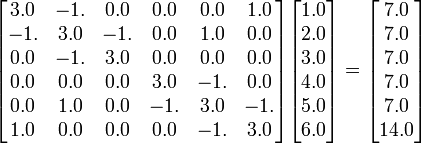Help Wiki

## SOFTWARE PACKAGES USAGE

### LAPACK

Solve Linear System Ax=b

As an example of using LAPACK let's consider the following identity of linear equations of order 6:$\mathbf \begin{bmatrix} 3.0 &-1. & 0.0 & 0.0 & 0.0 & 1.0 \\ -1. & 3.0 &-1. & 0.0 & 1.0 & 0.0 \\ 0.0 & -1. & 3.0 & 0.0 & 0.0 & 0.0 \\ 0.0 & 0.0 & 0.0 & 3.0 & -1. & 0.0 \\ 0.0 & 1.0 & 0.0 & -1. & 3.0 &-1. \\ 1.0 & 0.0 & 0.0 & 0.0 & -1. & 3.0 \end{bmatrix} \begin{bmatrix} 1.0 \\ 2.0 \\ 3.0 \\ 4.0 \\ 5.0 \\ 6.0 \\ \end{bmatrix} = \begin{bmatrix} 7.0 \\ 7.0 \\ 7.0 \\ 7.0 \\ 7.0 \\ 14.0 \\ \end{bmatrix}$

If we write the above identity with following symbols:

Ax = b

and assume that x is unknown, then we can try to solve for x using some LAPACK subroutine. Here is how we proceed:

• Find out what LAPACK subroutine solves the system of linear equations:
Ax = b
(subroutine dgesv is what we need).
• Write a fortran program which defines A, b and calls dgesv to solve for x
!  file_name = solve.f90

program solve_N6
!
!     This program solves Ax=B using LAPACK subroutine dgesv
!     A is a double precision 6 by 6 matrix defined in the program.
!     B is a double precision vector of length 6 also defined in the program.
!
implicit none
integer info, N, nrhs, lda, ldb
parameter (N=6, nrhs=1, lda=N, ldb=N)
double precision A(N,N), B(ldb,nrhs)
integer indx(N)

integer i, j
!
!     Defined matrix A:

data A / 3. , -1. ,  0. ,  0. ,  0. ,  1. ,                       &
&        -1. ,  3. , -1. ,  0. ,  1. ,  0. ,                       &
&         0. , -1. ,  3. ,  0. ,  0. ,  0. ,                       &
&         0. ,  0. ,  0. ,  3. , -1. ,  0. ,                       &
&         0. ,  1. ,  0. , -1. ,  3. , -1. ,                       &
&         1. ,  0. ,  0. ,  0. , -1. ,  3.      /

data B / 7. ,  7. ,  7. ,  7. ,  7. , 14. /
!
print *,"N = ", N
print *,"A = "
print *,A
print *,"B = "
print *,B
print *," "

call dgesv( N, nrhs, A, lda, indx, B, ldb, info )
!
!   Parameters of dgesv
!
!   N  is the matrix size, N >= 1 . (input)
!
!   nhrs is the number of Right Sides to be solved
!          --here 1 (input)
!
!    A is the matrix in Ax = B, overwritten on output by
!         L and U -- where L*U = A (rows permuted)
!         A is double precision N by N , but with leading
!         dimension lda >= N .
!         A is input, but is different on output (in/out) .
!
!    lda is the leading dimension of A.  lda >= N .
!
!    indx , integer array dimension N, indx(i)
!      was interchanged with the ith row of A.    (output)
!
!    B  , double precision array of dimension (ldb,nhrs)
!        On input it is the right hand side(s) B, on output
!        it is the solution vector(s) x.   (in/out)
!
!    info integer, output info = 0 indicates a successful exit
!                 info <= -i, the ith argument was illegal.
!                 info = i means u(i,i) was zero so factorization
!                    not completed.
!
print *,"X = "
print *,B

stop
end

• Write a makefile named Makefile to compile, link, and submit a job to the batch system
Make sure to include the ACML library for intel by issuing the command:
module load acml/ifort/4.4.0


before using the makefile Makefile:

# file_name = Makefile
# Makefile for solve.f90

ROOTDIR  = $(shell pwd) SRC = solve.f90 OBJ =$(SRC:.f90=.o)

LIBS = $(LDFLAGS) -lacml TARGET = solve_exec OUTPUTFILE=FORTRAN_LAPACK_SOLVE all:$(TARGET)

$(TARGET):$(OBJ)
$(FC) -o$@ $(CPPFLAGS)$(OBJ) $(LIBS)$(OBJ): ${SRC}$(FC) $(FFLAGS) -c${SRC}

help:;  @echo " "
@echo "USAGE: "
@echo "make help       To get this listing"
@echo "make            To compile the FORTRAN LAPACK program in current environment"
@echo "make subjob     To submit the executable to a batch queue using sqsub"
@echo "make clean      Remove *.o and executable files"
@echo "make list       List the compilers in current environment"
@echo " "

list:
@echo
@echo "ROOTDIR:     $(ROOTDIR)" @echo "FC Compiler:$(FC)"
@echo "FFLAGS:      $(FFLAGS)" @echo "LDFLAGS:$(LDFLAGS)"
@echo "OUTPUT FILE: $(OUTPUTFILE)" @echo " " @echo "LD_LIBRARY_PATH: "$(LD_LIBRARY_PATH)

clean:
@echo "Removing *.o and executable files"
rm -rf *.o $(TARGET) subjob:; rm -rf$(OUTPUTFILE)
sqsub -t -r 1h --mpp=2.0G  -o ${CLU}_$(OUTPUTFILE) -n 1 ./\$(TARGET)


For more details on the identity of linear equations of any order N, see:

Also, you can find more information on modules and make utility and makefiles in the following two references: